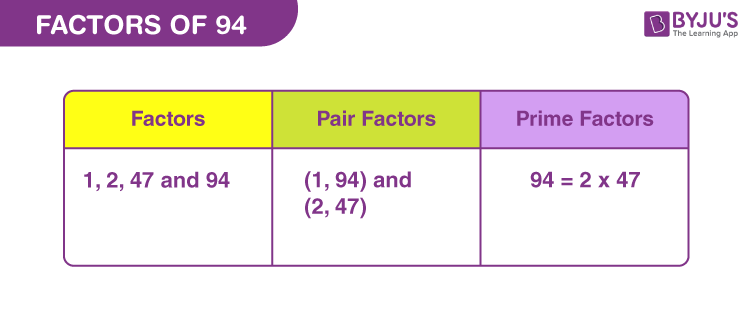# Factors of 94

In Mathematics, factors of 94 are the natural numbers that divide the original number uniformly. Alternatively, if the product of two whole numbers is equal to 94, then they are the required factors. Since 94 is a composite number, it has more than two factors. We can evaluate the factors by the simple division method. If the quotient obtained is a whole number and remainder is zero, then divisor is a factor.

Let us find the factors of 94 here in this article, along with pair factors and prime factors.## How to Find Factors of 94?

A factor is a component that breaks something in equal parts. By factorising, we mean, breaking a number in the form of a product of two or more numbers. Hence, 94 divided by its factors will be a whole number.

94 ÷ 1 = 94

94 ÷ 2 = 47

94 ÷ 47 = 2

94 ÷ 94 = 1

In all the above divisions we can see, the remainders are zero and quotients are the whole numbers. Thus, the factors of 94 are 1, 2, 47 and 94.

## Pair Factors of 94

When two numbers are multiplied and the result is 94, then the two numbers are the pair factors of 94 or called factors in pairs.

1 × 94 = 94

2 × 47 = 94

Therefore, the pair factors are (1, 94) and (2, 47).

In the same way, we can write the negative pair factors that will result in the original number, after multiplication.

-1 × -94 = 94

-2 × -47 = 94

Therefore, the negative pair factors are (-1, -94) and (-2, -47).

## Prime Factorisation of 94

Prime factors of 94 divide the original number into an equal number of parts. These prime factors are nothing but the prime numbers.

List of prime numbers (Between 1 and 94) :

 2, 3, 5, 7, 11, 13, 17, 19, 23, 29, 31, 37, 41, 43, 47, 53, 59, 61, 67, 71, 73, 79, 83, 89

Step 1: 94 is the even number. Thus, it is divisible by 2.

94/2 = 47

Step 2: Now from the list of prime numbers, we can see, 47 itself is a prime number, therefore,

47/47 = 1

Since, 1 is not divisible by any prime number. Therefore, the prime factors of 94 are 2 and 47.

 Prime factorisation of 94 = 2 x 47

## Solved Examples

Q.1: Find the sum of all the factors of 94.

Solution: The factors of 94 are 1, 2, 47 and 94 .

Sum = 1 + 2 + 47 + 94 = 144

Therefore, 144 is the required sum.

Q.2: What is the greatest common factor of 114 and 94?

Answer: To find the greatest common factors between two numbers, we need to list down the factors of each of them.

The factors of 114 are 1, 2, 3, 6, 19, 38, 57, 114

The factors of 94 are 1, 2, 61, 94

Then the greatest common factor is 2.

Q.3: What are the common factors of 94, 96 and 98?

Answer: Listing the factors of all the given numbers.

The factors of 94 are 1, 2, 47, 94

The factors of 96 are 1, 2, 3, 4, 6, 8, 12, 16, 24, 32, 48, 96

The factors of 98 are 1, 2, 7, 14, 49, 98

Therefore, Common factors are 1 and 2.

## Frequently Asked Questions on Factors of 94

### How many factors does 94 have?

There are a total of four factors of 94. These factors are 1, 2, 47, and 94.

### What are the prime factors of 94?

The prime factor form of 94 is: 2 x 47.

### What are the multiples of 94?

The first ten multiples of 94 are 94, 188, 282, 376, 470, 564, 658, 752, 846 and 940.

### How to find factors of 94?

Since 94 is an even number, thus it is divisible by 2. Now we need to check the product of which two numbers, results in 94. So, such pairs are (1, 94) and (2, 47). Hence, all the four numbers are the factors of 94.

### Is 94 a prime number or composite number?

94 is a composite number, since it has more than two factors, unlike prime numbers that have only two factors.

### Is 94 a perfect square?

No, 94 is not a perfect square because the root of 94 will not result in a natural number.
√94 = 9.695